# Surface area of a cone

The formula for the surface area of a cone is:

pir^2+pirl

Where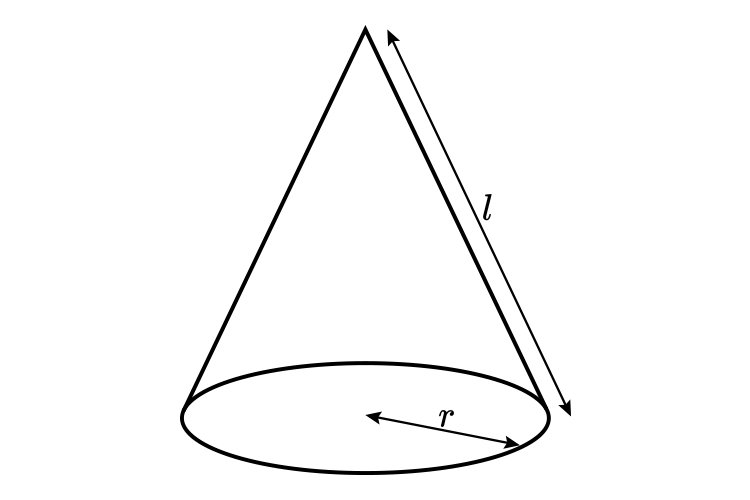This formula is easier to remember if you imagine pulling apart a cone to get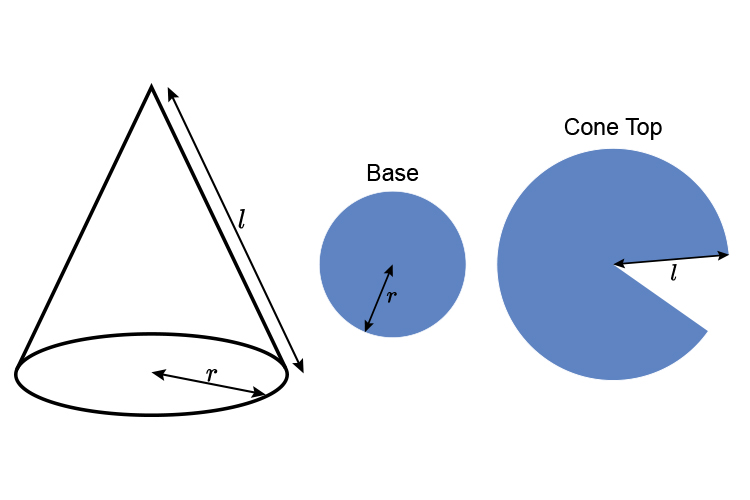The area of the base is: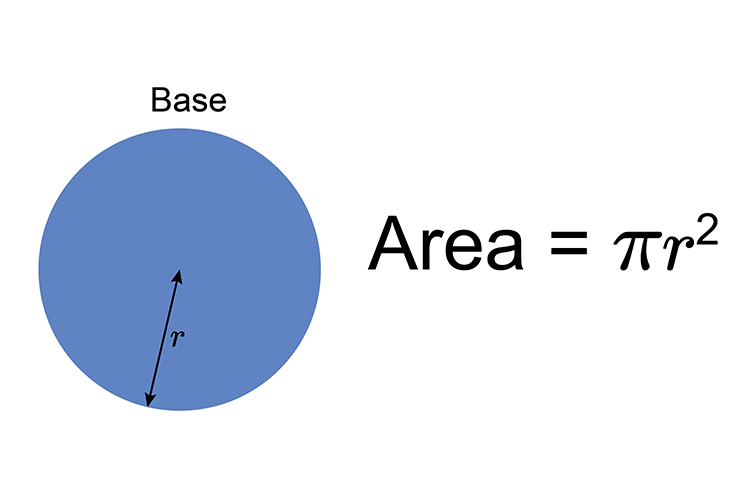The area of the cone top is: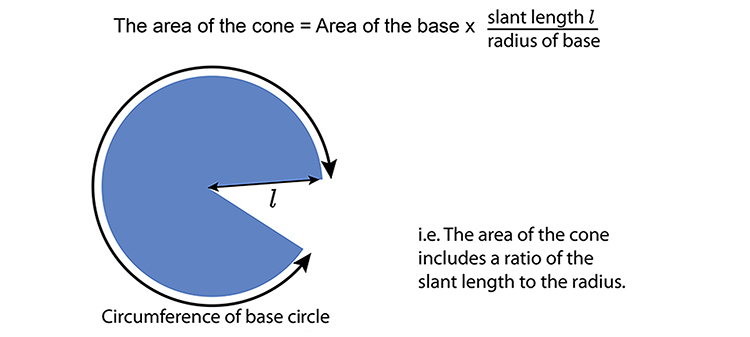The area of the cone top = Area base x ratio slant to radius

The area of the cone top =  \pir^2\timesratiol/r

The area of the cone top =   \pir^2\timesl/r

This goes to:

The area of the cone top =  \pirl

Summary

Area of a cone = Area of base + Area of cone top

=  \pir^2+\pirl

Interesting example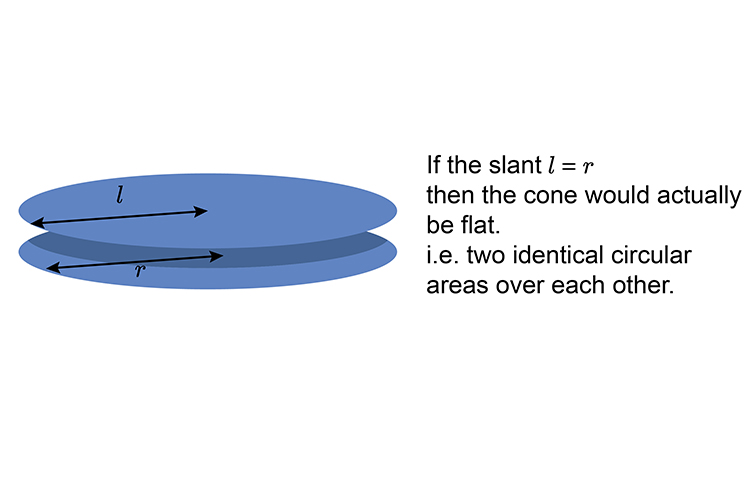And then pulling the cone apart the surface areas would look like this: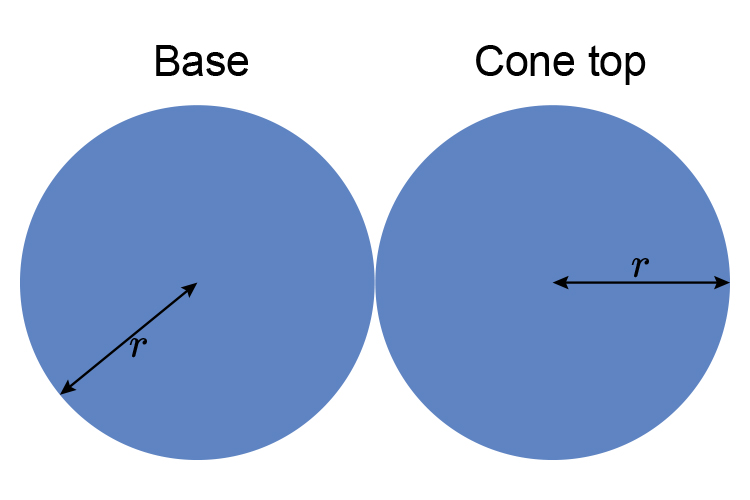That is two full areas of circles.

Or

\pir^2+\pir^2

Which becomes:  2\pir^2

So the surface area of a cone where the slant is the same as the radius is equal to the area of two base circles 2\pir^2

But this would never be a cone and therefore doesn’t exist under the heading “cones”.

## So the way to remember the surface area of a cone is to think:

\pir^2+\pir^2

## Only when the slant is equal to r

When it isn’t (which it can’t be as a cone).

Then the surface area of a cone must be:

\pir^2+\pirl